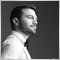• 概要
• レビュー (1)
• コメント

Pan PrizMA C D Phase

Pan PrizMA CD Phase is an analogue of the МetaТrader 5 indicator, and is an option based on the Pan PrizMA indicator. Details.

• Averaging by a quadric-quartic polynomial increases the smoothness of lines, adds momentum and rhythm.
• Extrapolation by the sinusoid function near a constant allows adjusting the delay or lead of signals.
• The value of the phase - wave status parameter (which is close in meaning to the trigonometric phase or the angle of the radius-vector rotation in the trigonometric plane) allows digitizing each line point and use any necessary point as a trading signal in the EA. This, in turn, accelerates optimization of strategies.
• Digitization allows you to set asymmetric permission/prohibition intervals during filtering. For instance, the interval prohibiting trading against a trend may comprise 90 degrees starting from any phase, only a quarter of the entire wave period.
• Using synthetic bars by volume, price or their combination allows adjusting the chart scale for the strategy.

External variables

• Line_1_power - degree of polynomial, averaging the first line (2-4).
• Fast_line_1_leverage - leverage (an analog of period) of a short average line smoothed by a polynomial.
• Fast_line_2_leverage - leverage of a short line extrapolated by the sinusoid near a constant.
• Base - half of the SMA period subtracted from the price. Analog of a Slow_SMA. The value of 0 excludes subtraction from the price.
• Synthetic_Channel - value of the synthetic bar defined as the sum of price points and the number of parts of the tick volume. The value of 0 disables synthetic bars.
• Weight_Prices - price multiplier. It allows changing the price value weight in the sum. The parameter can be negative.
• Weight_Volume - multiplier for the volume parts. It allows changing the volume value weight in the sum. The parameter can be negative.
• Multiplikator - histogram multiplier.
• Multiplikator_2 - price multiplier. -1 swaps the top and bottom lines.
• Signal SMA Period - signal line period.

Indicator lines

• "MACD_A0" - histogram. Plotted as the first difference on the extrapolated line. Read from the array 0.
• "Signal_A1" - histogram signal line. Read from the array 1.
• "Fast_line_1" - line averaged by the polynomial. Read from the array 2.
• "Fast_line_2" - extrapolated line. Read from the array 3.
• "Phase_A4" - phase line. Read from the array 4.
• "-_Phase_A5" - shifted phase line drawn below 0. It allows visualizing the first line on the bends. Read from the array 5.
• "+-2_Phase_A6" - dotted line provided by the MathArccos function converted from radians to degrees and multiplied by 2 to exclude coincidences with the phase lines. Read from the array 6.477
2019.01.29 05:55

Good Job